# HBSE 7th Class Maths Solutions Chapter 6 The Triangles and Its Properties Ex 6.5

Haryana State Board HBSE 7th Class Maths Solutions Chapter 6 The Triangles and Its Properties Ex 6.5 Textbook Exercise Questions and Answers.

## Haryana Board 7th Class Maths Solutions Chapter 6 The Triangles and Its Properties Exercise 6.5

Question 1.
PQR is a triangle right angled at P. If PQ = 10 cm and PR = 24 cm, find QR.
Solution: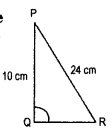(PR)2 = (PQ)2 + (QR)2
⇒ (QR)2 =(PR)2 – (PQ)2
= (24)2-(10)2
= 576 – 100 = 476
∴ QR = $$\sqrt{476}$$
= $$\sqrt{2 \times 2 \times 7 \times 17}=\sqrt{119}$$Question 2.
ABC is a triangle right angled at C. If AB = 25 cm and AC = 7 cm, find BC.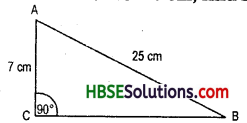Solution:
(AB)2 = (AC)2 + (BC)2
(25)2 = 72 + (BC)2
625-49 = (BC)2
576 = (BC)2
$$\sqrt{576}$$ =, BC
24 = BC
∴  BC = 24 cm.

Question 3.
A 15 m long ladder reached a window 12 m high from the ground on placing it against a wall at a distance (Fig. 6.56). Find the distance of the foot of the ladder from the wall.
Solution: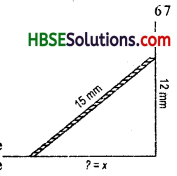Let ? = x
(15)2 = (12)2 + x2
225 -144 = x2
81 = x2
$$\sqrt{81}$$ = x
9 = x
∴  x = 9
The distance of the foot of the ladder from the wall = 9mm.Question 4.
Which of the following can be the sides of a right triangle ?
(i) 2.5 cm, 6.5 cm, 6 cm.
(ii) 2 cm, 2 cm, 5 cm.
(iii) 1.5 cm, 2 cm, 2.5 cm.
In the case of right angled triangles, identify the right angles.
Solution: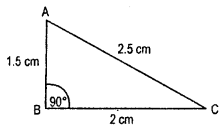In a right triangle
h2 = p2 + b2
(iii) (1.5 cm, 2 cm, 2.5 cm) is the right angle
h2 = p2 + b2
(2.5)2 = (1.5)2 + (2)2
6.25 = 2.25 + 4
6.25 = 6.25
This is satisfied.

Question 5.
A tree is broken at a height of 5 m from the ground and its top touches the ground at a distance of 12 m from the base of the tree. Find the original height of the tree.
Solution: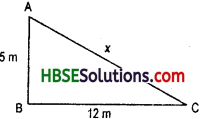Let the original height of tree = x
∴  x2 = (5)2 + (12)2
= 25 + 144 = 169
∴  x = $$\sqrt{169}$$
∴ The original height of the tree = 13 m.Question 6.
Angle Q and R of a APQR are 25° and 65°. Write which of the following is true:
(i) PQ2 + QR2 = RP2
(ii) PQ2 + RP2 = QR2
(iii) RP2 + QR2 = PQ2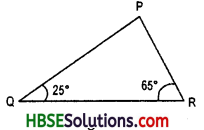Solution:
∠QPR = 180° – (25° + 65°)
= 180°-90°
∴  ∠QPR = 90
By Pythagoras Property,
(QR)2 = (PR)2 + (PQ)2
i.e. h2 = p2 + b2
hence, QR2 = (PQ)2 + (RP)2 i.e. (ii) is true.

Question 7.
Find the perimeter of the rectangle whose length is 40 cm and a diagonal is 41 cm.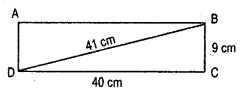Solultion:
From Fig 6.88
(BC) = (BD) – (CD)
= (41) – (40)
= 1681 – 1600 = 81
∴  BC =  = 9 cm.
Thus the perimeter of the rectangle
= 2 ( l x b) = 2(40 + 9)
= 2 x 49 = 98 cm.Question 8.
The diagonals of a rhombus measure 16 cm and 30 cm. Find its perimeter.
Solution: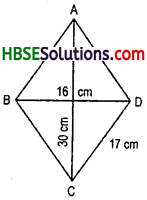Area of Rhombus
= $$\frac{1}{2}$$ x d1 x d2 Sq.units.

(where d1 and d2 are diagonals of rhombus) Side of Rhombus (a) = $$\frac{1}{2} \sqrt{\left(d_{1}\right)^{2}+\left(d_{2}\right)^{2}}$$
Perimeter of Rhombus = 4 x a
Here,
d1 = 16 cm
d2 = 30 cm
∴  Side of Rhombus (a)
= $$\frac{1}{2} \times \sqrt{(16)^{2}+(30)^{2}}=\frac{1}{2} \times \sqrt{256 \times 900}$$
= $$\frac{1}{2}$$ x $$\sqrt{(16)^{2}+(30)^{2}}$$ = $$\frac{1}{2}$$ x 34 =17 cm z z
∴ Perimeter of Rhombuse
= 4 x a = 4 x 17= 68
∴ Perimeter of Rhombus = 68 cm.